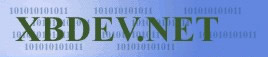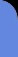Saturday July 4, 2020
 home | about | contact | Donations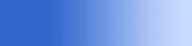The Maths of 3D You can't have 3D without a little maths...

Rasterization : Drawing Lines

by bkenwright@xbdev.net

When many people today start out in graphics they take things like Line drawing algorithms for granted...or Triangle Fill API's etc in the DirectX library's...with little or no understanding of how to implement a version themselves.  And as you can guess....that's what where going to do here today.  Where going to analyse how you'd draw a line to the screen...taking into account optimisation and speed considerations.

Of course we'll still be using DirectX for the screen, so we can access the screen pixels directly... but we'll be doing everything else ourselves.

A line, what is it?  Well its two points... a source point...and a destination point...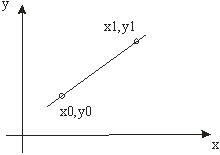where y=mx +c is the equation of a line that where taught at school

Or alternatively put,

y = y0 + t*(y1-y0)

x = x0 + t*(x1-x0)

where t goes from 0 to 1 (usually represented as t => 0:1 )

But to the point...if we start at (x0,y0), then we can increment our changes using the gradient... as show:

 // int x0, y0 - start point       // int x1, y1 - end point             float dy = y1-y0;       float dx = x1-x0;       float m = dy/dx;         int y=y0;       for(int x=x0; x

That would be our simple approximation...and would work... but there is one more thing in the world of computer code.... the y value of 0 is at the top of the screen...and increments downwards.

 DownloadSourceCode void Render(unsigned int* pBits, int w, int h, int pitch) {       clearscreen(pBits, w, h, pitch);           int x0 = 100; // start point       int y0 = 100;         int x1 = 200; // end point       int y1 = 200;             float dy = y1-y0;       float dx = x1-x0;       float m = dy/dx;         float y=y0;       for(int x=x0; x

So what we have is this...we increment x by 1 at a time....and we increment y by the gradient m.....m = ( y1-y0) / (x1-x0)

Now the big big problem with the above code, which will be mentioned in nearly every optimisation book, is the conversion of float to int....notice the type casting in the setpixel(..) function call.  As each time through the loop we need to convert our float to a int to plot that pixel... now it may not seem like much of a penatly ...but just you wait and see...when you start rendering millions of triangles it soon slows down.

But there is a number of solutions... the first is an approximation of the Bresenham Algorithm,... which uses the theory of how we add fractions... as if you notice how we do this in our inner loop:

y += m

which is the same as:

y += dy/dx

or

y += (y1-y0)/(x1-x0)

If we note that when we add fractions, which the same denominator...e.g. the same bottom divider amount...we only need to add the top numerator values...and if it is greater than the denominator...we can just subtract it... ..let me give you a simple example with numbers:

y  =  y + dy/dx                               = y + dy/dx

y  =  y + dy/dx + dy/dx                  = y + 2* dy/dx

y  =  y + dy/dx + dy/dx + dy/dx     = y + 3* dy/dx

Well I know I know..its a terrible example...I can only hope you get a piece of paper out and dig the principle into your head...as you'd be amazed how many times it pops up.  So using this simple idea, we can modify our line drawing algorithms

 DownloadSourceCode void line(unsigned int* pBits, int w, int h, int pitch,               int x0, int y0,               int x1, int y1,               DWORD dwColour) {       float dy = (float)y1-y0;       float dx = (float)x1-x0;       float m = dy/dx;         float ynum = dy;       float yden = dx;       int   y = y0;         for(int x=x0; x= dx )             {                   ynum -= dx;                   y++;             }       }// End for loop x   }// End of line(..)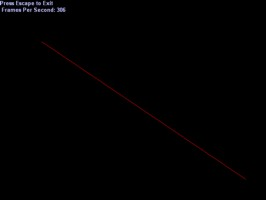But there is one more little thing which I've left out up until now, as I didn't want to put to much information on you at once...its whether you should increment x or y as the outer loop...if that makes any sense?

Let me give some examples as I try explain to those who are new to this:

( dx > dy )

given:  p0 = (2, 1) and p1 = (10, 2 )

...now dx = x1-x0 = 10 - 2 = 8

dy = y1-y0  = 2 - 1  = 1

And our gradient m = dy/dx = 1/8

So if we increment x from x0 to x1  we go: 2, 3, 4, 5, 6, 7, 8... and we do the same for y... we get 1, 1.125, 1.25, 1.375... etc to 2.   But remember those decimal values are rounded off to integers...so we get something like, 1,1,1,1, 2,2,2,2 for the y values :)

Well comes this next case:

(dy > dx)

say: p0=(2,1) and p1 = (3,6)

So we calcuate our dx and dy values, which come to:

dx = 1, dy = 5

Start x at x0, then increment it, and we have x1....which is a single step...... 2, 3..... now do the same for y and we get 1,6  ... ackkk...a jump from 1 to 6?... that can't look good....I'll show you a sketch of what it looks like:Well its better if you do a small sketch on a pad or something... but the idea is, we determine to increment x or y in the outer loop depending on whether dx or dy is largest.... and alter the gradient appropriately...so our function code now becomes:

 DownloadSourceCode void line(unsigned int* pBits, int w, int h, int pitch,               int x0, int y0,               int x1, int y1,               DWORD dwColour) {       float dy = (float)y1-y0;       float dx = (float)x1-x0;       float m = dy/dx;         if( dx > dy )       {             float ynum = dy;             int y = y0;             for(int x=x0; x= dx )                   {                         ynum -= dx;                         y++;                   }             }// End for loop x       }       else       {             float xnum = dx;             int x = x0;             for(int y=y0; y= dy )                   {                         xnum -= dy;                         x++;                   }             }// End for loop y       }   }// End of line(..)Well I guess it looks like a lot... but its really not that much... you can probably see if better if I did it like this:

if( dx > dy )

x++;

y+=dy/dx;

else

y++

x+=dx/dy;

All that Besenham code makes things look messy and complex...also I've layed it out in a simple to understand manner I hope...

The final straw, before moving on with our line algorithm, is deciding if x0 is greater or less than x1..... as we can't rely on a person using our line algorithm to use is by passing in the coordinates in a specific order... so we must check for this and account for it!  Always best to be safe.

 DownloadSourceCode void line(unsigned int* pBits, int w, int h, int pitch,               int x0, int y0,               int x1, int y1,               DWORD dwColour) {       int dy = y1-y0;       int dx = x1-x0;         int sdx = ( dx<0 ) ? -1 : 1 ; // sign of dx and dy       int sdy = ( dy<0 ) ? -1 : 1 ;         dx = dx * sdx;       dy = dy * sdy;         int px = x0; // starting point (x0,y0)       int py = y0;           if( dx > dy )       {             int y=0;             for(int x=0; x= dx )                   {                         y -= dx;                         py += sdy;                   }                   px += sdx;               }// End for loop x       }       else       {             int x=0;             for(int y=0; y= dy )                   {                         x -= dy;                         px+= sdx;                   }                   py+= sdy;             }// End for loop y       }   }// End of line(..)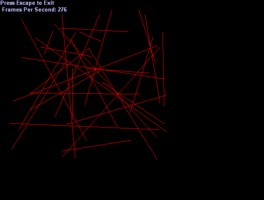Well thats a pretty good method of drawing a line I think... but there's more than one way to skin a cat as they say.  When I first learned that method, I thought it was the best...but with a bit of creativity, you can do a line drawing algorithm using 'Fixed Point Maths'

It takes a bit of getting used to at first, but you'll soon come around believe me....

So taking an approximation of our line drawing algorithm using real numbers, it would be:

 if( dx > dy ) {             for(x=0; x

Let me see....hmmm....a popular Fixed Point value is 16:16...where 16 bit's are for the whole number part, and 16 bits for the decimal part....giving us:

#define FIXEDPOINT_16_16 16

So to convert a integer to a fixed point value we do this:

int iFPV = i << FIXEDPOINT_16_16;

Where iFPV is our new fixed point value.... So I re-wrote our line drawing algorithm to use fixed point numbers....if you get stuck...don't worry...just try some simple examples....things like this:

a = 10;

b = 33;

A = a << FIXEDPOINT_16_16; // Convert to fixed point integer

B = b << FIXEDPOINT_16_16;

C = A+B;

c = C >> FIXEDPOINT_16_16;  // Convert back to standard integer

Few things to watch out for, when playing around with fixed point numbers, is sign...as the upper most number with signed integers is used to represent the sign of our value...so don't go converting negative values to Fixed Point values without care...as it will give some crazy results.

 DownloadSourceCode void line(unsigned int* pBits, int w, int h, int pitch,               int x0, int y0,               int x1, int y1,               DWORD dwColour) {        int dy = (y1-y0);       int dx = (x1-x0);         int sdx = ( dx<0 ) ? -1 : 1 ; // sign of dx and dy       int sdy = ( dy<0 ) ? -1 : 1 ;         dx = dx * sdx;       dy = dy * sdy;         int px = x0 << FIXEDPOINT_16_16; // starting point (x0,y0)       int py = y0 << FIXEDPOINT_16_16;         // Should check for divide by zero errors here.       int dxdy = 0;       if( dy!=0 ) dxdy = ((dx << FIXEDPOINT_16_16)/dy)*sdx;         int dydx = 0;       if( dx!=0 ) dydx = ((dy << FIXEDPOINT_16_16)/dx)*sdy;         sdx = (1 << FIXEDPOINT_16_16)*sdx;       sdy = (1 << FIXEDPOINT_16_16)*sdy;           if( dx > dy )       {             for(int x=0; x>FIXEDPOINT_16_16,                               py>>FIXEDPOINT_16_16, dwColour);                                     py += dydx;                   px += sdx;                }// End for loop x       }       else       {             for(int y=0; y>FIXEDPOINT_16_16,                               py>>FIXEDPOINT_16_16, dwColour);                                     px += dxdy;                   py += sdy;               }// End for loop y       }   }// End of line(..)I know ...I know....thats a lot of code...and it looks messy!....eekkKKkk... The algorithm has reached a peak in performance I think...  I didn't want you to think that you had done all this work and not got anything to show for it, so I started up VTune from intel, which gives some performance details ... and it showed quite a difference.

For the Beszenier Line algorithm (code_4), it was an average of 1,567 clock cycles for the function....for our new improved fixed point one, it came out to be 307!  Wow!.. thats not bad...well I thought it was okay.

We could make the SetPixel(..) function inline as well...to make it a bit better I guess.... but why the performance difference was so great?  Well putting anything in a loop is bad!  And those if statements cost a lot more than a simple binary shift...as a simple example was "if(y>=dx)" was cosing 145 clock cycles approx....

A careful think to look out for is simple "overflow" or "out by one errors" when your developing or changing the code...its well worth single stepping through it and checking the values :)

 Visitor: 9534626  { 209.237.238.175 } Copyright (c) 2002-2020 xbdev.net - All rights reserved. Designated tutorial and software are the property of their respective owners.